[Next] [Up] [Previous]

# Co-ordinates and Distances

If one desires to program a computer to draw a picture of a Platonic or an Archimedean solid, one needs to know where its vertices are located, or the angles of its faces. How might one determine that?

For the cube and the octahedron, at least if the simplest possible orientation is chosen, the answer is obvious. For a cube with a unit edge, the vertices are at x = +/- 1/2, y = +/- 1/2, z = +/1 1/2, with the three quantities varied independently. For an octahedron with a unit edge, two of the vertices are x = +/- 1/sqrt(2), y=0, z=0, and the other four are the same thing, but with either y or z being the nonzero coordinate.

Simple addition allows one to proceed from these to the vertices for the small rhombicuboctahedron, and the great rhombicuboctahedron as well as the cuboctahedron, are only alightly more difficult, and may safely be left as an exercise for the reader.

To handle the tetrahedron, one has to rembember that in one orientation, its vertices are four of the eight vertices of the cube. Once that is realized, that the vertices of a tetrahedron with unit side, in a simple orientation, are x = - 1/(2 sqrt(2)), y = - 1/(2 sqrt(2)), z = 1/(2 sqrt(2)), and the same with every other combination of zero or two minus signs jumps out immediately.

But what about the dodecahedron and the icosahedron? With those, it is hard even to tell where to begin!

But we've seen before that the dodecahedron contains, hidden within it, the symmetry of the cube. That is a good starting point. And there is one other thing we can use: the power of the golden ratio.

The golden ratio, phi, has the property that 1 + 1/phi = phi. Therefore, phi^2 - phi - 1 = 0, and substituting into (-b + sqrt(b^2 - 4*a*c))/(2a), the formula for one of the roots of the quadratic equation, yields (1 + sqrt(5))/2, which approximately equals 1.6180339887498948482.

It also happens to be useful in the geometric construction of a pentagon, as it happens that the ratio between the length of a line joining two nonadjacent corners of a pentagon and the length of a side of the pentagon is phi. Since a line phi times another line in length can be constructed with straightedge and compasses (a line twice as long as another line can be constructed, from which the Pythagorean theorem makes it easy to construct a line with the square root of 5 as its length), it is possible to construct a pentagon with straightedge and compasses used in conventional fashion. (Using them in unconventional fashion, one can trisect the angle, as the ancient Greeks knew, but while creative use of tools can be useful, the mathematical proofs of what can and can't be done using only the conventional straightedge-and-compass operations have also been very useful to the development of mathematics.)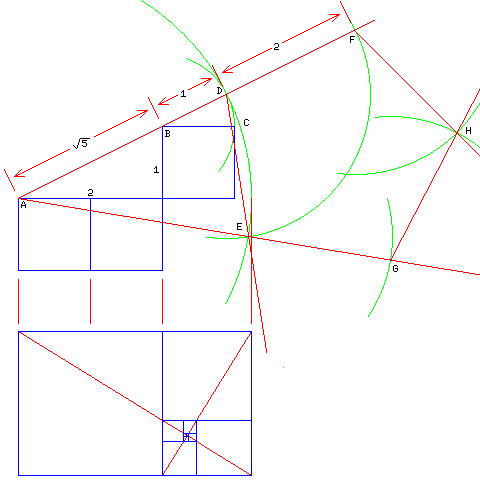The diagram above illustrates the geometrical construction of a regular pentagon, and the role the Golden Ratio plays in that construction. Three settings of the compass are used, first to a radius of 1, then to a radius of sqrt(5) + 1, and finally, several times, to a radius of 2, and the pentagon constructed has a side of 2.

The line from A to B has the length of the square root of 5 by the Pythagorean theorem. Extending that line, and placing the point of the compass at B, and drawing a circle whose radius is unity, as, for example, exemplified by the distance from B to C, lets us place point D on the line at a distance of sqrt(5) + 1, which is twice the Golden Ratio. Placing the point of the compass at A, and drawing an arc from D, and then placing the point of the compass at D, and drawing a circle with a radius of two, we obtain, at point E, the intersection of the two circles, a second point on a pentagon whose side is 2, which is in a pentagram one of whose points is at A.

Continuing both lines AD and AE, and drawing more arcs of length 2, we can obtain two more of the corners of the pentagon, F and G, and then using both of those points as the center of a circle of radius 2, at the intersection of the arcs we can find the point H which completes the pentagon.

Incidentally, though, you may be wondering why the pentagon has this property connected with the Golden Ratio. The diagram below may make an explanation of this intelligible.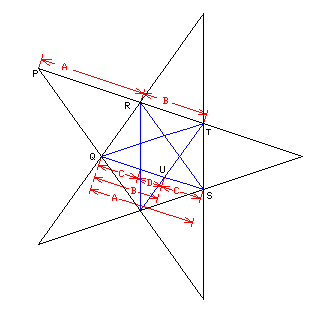From symmetry, one can immediately see that, in this diagram of a regular pentagram with another regular pentagram embedded in it, that the line PR is parallel to the line QS.

Similarly, the line PQ must be parallel to the line RS.

This means that the length of the line segment PR, which is called length A, is also the length of the line segment QS. Thus, the ratio between the length of a side of a pentagon (i.e., line segment RT) to both a line joining two nonadjacent corners of the pentagon (i.e., line segment QS) is the same as the ratio of that length to a line from a corner of the pentagon to an adjacent point on the star of which it is the center (i.e., line segment PR).

Again, since both RQ and TU are parallel, and RT and QU are parallel, the distance from R to T, called B, is also the distance from Q to U.

Since we are dealing with two similar shapes, a large pentagram and a smaller one inside it, we know that length C is to length D as length A is to length B. Therefore, we now have some matching ratios of lengths available to us.

``` A     C     C + D + C
--- = --- = ----------- = x
B     D       C + D
```

Given that C/D = x, we can substitute this fact into the third ratio shown, which is A/B, but with A and B divided up into pieces of length C and D, to obtain:

```      C
C + --- + C
x
------------- = x
C
C + ---
x
```

We now can divide C out of the top and bottom to learn about x, and we find that

``` 2 + 1/x
--------- = x
1 + 1/x
```

and, cross-multiplying, this means that 2 + 1/x = x + 1, so 1 + 1/x = x, the defining property of the Golden Ratio noted above.

Once we know this, we can also determine that B/C is equal to the Golden Ratio as well.

Applying this knowledge to locating the vertices of a dodecahedron is depicted by the diagram below.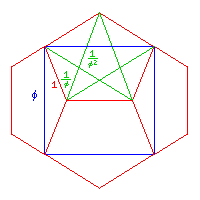With the dodecahedron in the orientation required for the simplest use of the cube within, eight of its twenty vertices are located immediately: they are at x = +/- phi/2, y = +/- phi/2, z = +/- phi/2 for all eight possible combinations of signs, because for a dodecahedron of side 1, the cube has side phi.

The remaining twelve points are all very symmetrical, and so it is only necessary to locate one of them.

The point we will locate is the one to the left of the center of the image. We will choose the co-ordinate representation in which the z axis points left, the y axis points up, and the x axis points towards the viewer.

This point is clearly at z = 1/2 and y = 0. It is its x coordinate that we must derive.

The pentagram drawn on the pentagon in the top of the diagram is, of course, foreshortened, as the pentagon is tilted away from the viewer. A similar pentagon may be drawn on any of the other pentagonal faces of the dodecahedron. The topmost point of the star is an isosceles triangle whose two sides of the same length have length 1/phi, and whose base has side 1/(phi*phi). The height of this triangle can, therefore, be calculated from the Pythagorean theorem, and is sqrt( (1/phi)^2 - (1/(2*phi*phi))^2 ), which equals (1/(2*phi*phi)) * sqrt( 4*(phi^2) - 1 ).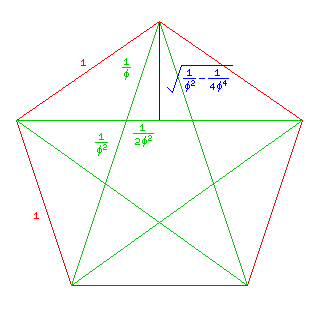Substituting the definition of phi, (1 + sqrt(5))/2, we obtain (1/(2*phi*phi)) * sqrt( 5 + 2 * sqrt(5) ) as the height of this triangle.

The height of this triangle is also the length of a line connecting the midpoint of the left front edge of the cube (at y = 0, x = phi/2, z = phi/2) and the point whose location we wish to derive. It is the hypotenuse of a right triangle, and one of its other sides has the length phi/2 - 1/2, corresponding to the difference in z coordinates between the point and the left face of the cube. The length of the other side will give the difference in x coordinates between the point and the front face of the cube.

It is possible to calculate the desired coordinate in a more straightforwards manner, however. Looking at the slanted line on the left of the star on the top front face of the dodecahedron, we see not only a line segment of length 1/phi on the top part, but two line segments, of lengths 1/phi and 1/(phi*phi) on the bottom part. Added together, those two line segments have length 1. So the height of the top part of the pentagon is in the ratio of 1/phi to the height of the bottom part of the pentagon.

Incidentally, the height of the pentagon as a whole is, therefore, phi^2 times the height of the top triangle, therefore, or 1/2 * sqrt( 5 + 2 * sqrt(5) ).

How do we make use of the ratio between the heights of the top and bottom parts of the pentagon?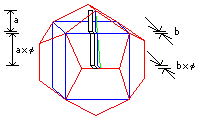Let us temporarily shift to considering another one of the vertices of the dodecahedron under consideration, the one on the top of the top front pentagon. It is at z = 0 and x = 1/2, with its y coordinate being the same unknown number we are currently seeking.

The distance along the x axis between this point and the edge of the cube within the top front pentagon is phi/2 - 1/2.

If that distance is then multiplied by phi, which is the ratio between the heights of the top and bottom parts of that pentagon, we now get the distance along the x axis between the original point under consideration and the front of the cube: (phi^2)/2 - phi/2. (Incidentally, that is 1/2 * ((phi^2) - phi), which is 1/2 * phi * (phi-1), and, since phi-1 = 1/phi, that simplifies to 1/2.) Adding phi/2, the x coordinate of the front of the cube, gives that point's x coordinate, (phi^2)/2, which is also (1 + phi)/2.

Incidentally, from the points on the vertices of the cube, we know that the distance from any vertex of the dodecahedron with a unit side to the center of the dodecahedron is sqrt(3) * phi/2, which is approximately 1.401258538444.

Since the icosahedron is the dual of the dodecahedron, we can produce a brute-force solution simply by taking the co-ordinates of the five vertices of a dodecahedron which are also at the corners of a particular one of its pentagonal faces, and averaging them to obtain the co-ordinates of the point at the center of the face.

But we can do better than this. Both the dodecahedron and the icosahedron have 30 edges. Not only do they both have the same number of edges, in one sense they have the same edges, as the diagram below illustrates: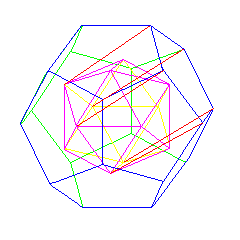Since the icosahedron is the dual of the dodecahedron, its vertices point in the same direction from its center as the faces of the dodecahedron point, and the five triangles around a vertex form a pentagon which lies in the same plane as the corresponding face of the dodecahedron. However, that pentagon is in the opposite orientation, and so one has to continue to the other side of the icosahedron to find the matching pentagon.

The diagram below shows how the relationship of both the dodecahedron and the icosahedron to the rhombic triacontahedron, and the fact that the faces of the rhombic triacontahedron belong to families that resemble the cube, mean that the edges of both these Platonic solids have directions that are normals to the faces of the rhombic triacontahedron.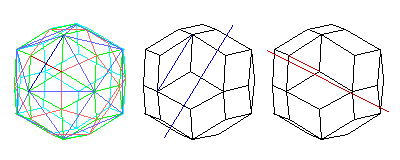This, therefore, explains how the pegs stuck into holes of the square faces of the small rhombicosidodecahedron, which have the same orientations as the faces of the rhombic triacontahedron, can be used to construct not only the icosahedron and dodecahedron, but also those other Archimedean solids all of whose edges adjoin at least one face which corresponds in orientation to the faces of either the icosahedron or the dodecahedron. A hexagonal face corresponds to two opposed triangles, and a decagonal face corresponds to two opposed pentagons, so these faces are permitted as well.

The list of Archimedean solids that can be constructed in this way for that reason is:

```truncated dodecahedron
icosidodecahedron
truncated icosahedron
small rhombicosidodecahedron
great rhombicosidodecahedron
```

In addition to those Archimedean solids, it is clear that the cube can also be constructed this way.

If one works with a connector in the shape of the small rhombicosidodecahedron rather than the rhombic triacontahedron, one gains in addition the ability to insert connectng rods from a connector at the center of either an icosahedron or a dodecahedron to its vertices. These rods will be different in length from those used for other directions.

Note that because the icosidodecahedron is the dual of the rhombic triacontahedron, connecting rods from a connector at its center to its vertices are also possible, in this case emanating from the square faces of the small rhombicosidodecahedron.

This principle, along with a design of connecting rods that ensures that each rod is used in only the right direction, and every connector ball is in the same orientation, is the basis of the geometrical model set mentioned previously: the Zome System.

As noted, to make the triangular faces more equal in area to the pentagonal faces, and to control the orientation of connector rods inserted in the now rectangular faces of the resulting connector, the square faces of the small rhombicosidodecahedron are replaced by rectangles, which happen to be Golden Rectangles.

Connector rods inserted into the rectangular faces are blue in color; they have effective length (the distance between the centers of the connectors which they create) 1 and phi (and so on).

Connector rods inserted into the triangular faces are yellow in color: they have effective length sqrt(3)/2, sqrt(3) * phi/2, sqrt(3) * (phi^2)/2 (and so on).

Connector rods inserted into the pentagonal faces are red in color.

And to determine their effective lengths, we need to use the fact we have noted about the edges of the icosahedron to determine the coordinates of its vertices, so that we will know the distance from the center of an icosahedron to its vertices.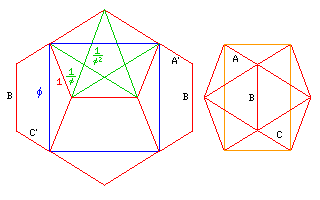The orientation of the dodecahedron and icosahedron shown here causes the edges on the back of each solid to be hidden; thus, on the dodecahedron, edges A' and C' are labelled, which are directly in front of the edges which genuinely correspond to edges A and C of the icosahedron, and which differ from the desired edge by having an opposing displacement in the direction towards the viewer (which had been designated the x direction in the discussion above).

The displacement between the vertex chosen as the starting point in the diagram above and the center of the icosahedron is now clear: take the y and z displacements only of edge A, and add a y displacement of 1/2, for half the length of edge B.

And we can do even better, since looking at the blue square drawn on the dodecahedron on the left shows us that a Golden Rectangle is defined by four of the vertices of the icosahedron in this orientation. Thus, the point is clearly at z = 1/2, y = phi/2.

Its distance from the origin is therefore sqrt(1/4 + (phi^2)/4), which is approximately 0.951056516295.

Using what we have calculated up to this point to determine the coordinate displacements for a unit vector in each of the possible directions normal to the faces of the rhombic triacontahedron, the underlying simplicity of the symmetries of the subset of the Archimedean and Platonic solids under examination, and their relationship to the Golden Ratio, becomes obvious: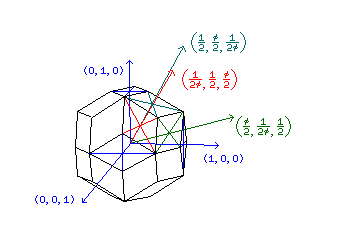These are also the vertices of an icosidodecahedron, since that is the dual of the rhombic triacontahedron; as it happens, the side of that icosidodecahedron is 1/phi, so multiplying each point by phi produces the vertices of a icosidodecahedron with a unit side.

Why is that? The icosidodecahedron can be divided into halves along several planes into two domes whose base is a regular decagon, so it is sufficient to demonstrate that the sine of 18 degrees must be phi/2, or that the distance from the center of a regular decagon to its corners is phi times the side of the decagon.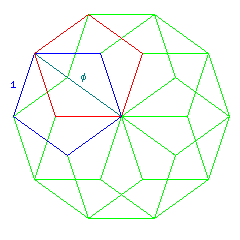We can see this because one way to construct a decagon is to alternate between opposing faces of a pentagon. This creates a pattern in which we see that the pentagons must all have one of their corners at the center of the decagon, because a path involving two of the sides from each successive pentagon leads to the same point as a path involving another two of the sides from the next one, and this path has a length of phi times the side of the pentagon, which is also the side of the decagon.

Starting from the icosahedron, the dodecahedron, and the icosidodecahedron, we can then use relationships between these solids and the remaining solids in this group to derive their coordinates.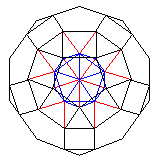If we enclose an icosahedron with a small rhombicosidodecahedron, we can draw triangular prisms from each face of the icosahedron to the corresponding triangular faces of the small rhombicosidodecahedron. Edges of these prisms also form edges of pentagonal prisms issuing from the vertices of the icosahedron.

Because the edges of a prism are parallel, and because the pentagonal faces of the small rhombicosidodecahedron are in the same orientation as those of a dodecahedron, the height of the triangular prisms equals the distance between the vertices of a dodecahedron and its center.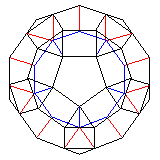Similarly, if we put a dodecahedron at the center of a small rhombicosidodecahedron, we can create pentagonal prisms from the faces of the dodecahedron to the corresponding pentagonal faces of the small rhombicosidodecahedron. Again, the edges that lead from one pentagon to the other are parallel, and the triangular faces are oriented in the same direction as those on an icosahedron, and so the triangular pyramids which join the triangular faces of the small rhombicosidodecahedron to the vertices of the dodecahedron could, if fitted together by themselves, construct an icosahedron.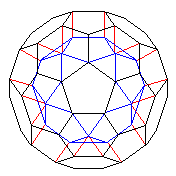We can also construct pentagonal prisms joining corresponding pentagonal faces of a truncated icosahedron and an icosidodecahedron that it encloses. What can we deduce considering the height of those prisms?

If we split an equilateral triangle into three shallow triangles, each with an apex at the center of the triangle, and we move these triangles out so that the sides of the original triangle now touch alternating sides of a hexagon, we find that the other sides are parallel.

This means that we once again have prisms the height of which matches the distance of the vertices of an icosahedron from its center, because the triangular pyramids which make an icosahedron have simply been split into three pieces.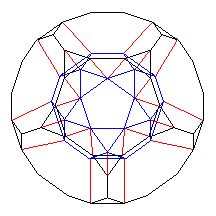When a truncated dodecahedron encloses an icosidodecahedron, this time we can make triangular prisms. And the change from a pentagon to a decagon follows the same rule as the change from a triangle to a hexagon: so the height of the triangular prisms is again the distance from the vertices of a dodecahedron to its center.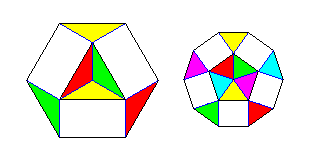This diagram illustrates why going from a point to a triangle is like going from a triangle to a hexagon, and why going from a point to a pentagon is similarly like going from a pentagon to a decagon; as noted, one can think of splitting the triangle into three pieces, or the pentagon into five, and what remains of the area added going from one shape to the next has parallel sides.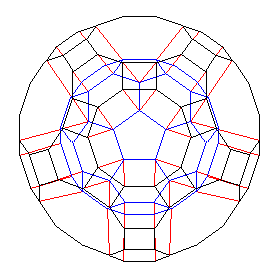And finally we deal with the great rhombicosidodecahedron.

We can form a small table:

```small rhombicosidodecahedron = icosahedron + dodecahedron
truncated icosahedron =        icosahedron +                icosidodecahedron
truncated dodecahedron =                     dodecahedron + icosidodecahedron
great rhombicosidodecahedron = icosahedron + dodecahedron + icosidodecahedron
```

and, just as we constructed the small rhombicosidodecahedron either by starting with the icosahedron, and moving out with triangular prisms whose height was the distance of the vertices of a dodecahedron from its center, or by starting with the dodecahedron, and moving out with pentagonal prisms whose height was the distance of the vertices of an icosahedron from its center, we can, as is done in the diagram above, start with the truncated icosahedron and use hexagonal prisms whose height is the distance of the vertices of a dodecahedron from its center, or we can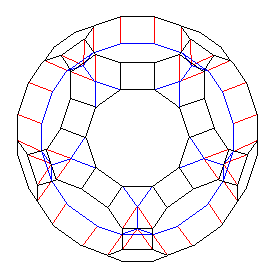start with the truncated dodecahedron and use decagonal prisms whose height is the distance of the vertices of an icosahedron from its center.

Here is a listing of the vertices of the solids we are considering, in this particular choice of a simple orientation (signs for each +/- in a row are independent, and run over all possible values) in order of size, from smallest to largest:

```             x                            y                            z

Cube (8 vertices)
+/- 1/2                      +/- 1/2                      +/- 1/2

Icosahedron (12 vertices)
0                            +/- phi/2                    +/- 1/2
+/- phi/2                    +/- 1/2                      0
+/- 1/2                      0                            +/- phi/2

Dodecahedron (20 vertices)
+/- phi/2                    +/- phi/2                    +/- phi/2
+/- 1/2                      +/- (phi^2)/2                0
+/- (phi^2)/2                0                            +/- 1/2
0                            +/- 1/2                      +/- (phi^2)/2

Icosidodecahedron (30 vertices)
+/- phi                      0                            0
0                            +/- phi                      0
0                            0                            +/- phi
+/- phi/2                    +/- (phi^2)/2                +/- 1/2
+/- (phi^2)/2                +/- 1/2                      +/- phi/2
+/- 1/2                      +/- phi/2                    +/- (phi^2)/2

Small Rhombicosidodecahedron (60 vertices)
+/- (phi^3)/2                +/- 1/2                      +/- 1/2
+/- 1/2                      +/- (phi^3)/2                +/- 1/2
+/- 1/2                      +/- 1/2                      +/- (phi^3)/2
+/- (phi^2)/2                +/- phi/2                    +/- phi
+/- phi/2                    +/- phi                      +/- (phi^2)/2
+/- phi                      +/- (phi^2)/2                +/- phi/2
+/- (phi^2)/2                +/- (phi^2 + 1)/2            0
+/- (phi^2 + 1)/2            0                            +/- (phi^2)/2
0                            +/- (phi^2)/2                +/- (phi^2 + 1)/2

Truncated Icosahedron (60 vertices)
+/- (phi^3)/2                +/- 1                        +/- phi/2
+/- 1                        +/- phi/2                    +/- (phi^3)/2
+/- phi/2                    +/- (phi^3)/2                +/- 1
+/- (phi^2 + 1)/2            +/- 1/2                      +/- phi
+/- 1/2                      +/- phi                      +/- (phi^2 + 1)/2
+/- phi                      +/- (phi^2 + 1)/2            +/- 1/2
+/- 3*phi/2                  +/- 1/2                      0
+/- 1/2                      0                            +/- 3*phi/2
0                            +/- 3*phi/2                  +/- 1/2

Truncated Dodecahedron (60 vertices)
+/- (phi^3)/2                +/- (phi^2)/2                +/- phi
+/- (phi^2)/2                +/- phi                      +/- (phi^3)/2
+/- phi                      +/- (phi^3)/2                +/- (phi^2)/2
+/- phi^2                    +/- 1/2                      +/- (phi^2)/2
+/- 1/2                      +/- (phi^2)/2                +/- phi^2
+/- (phi^2)/2                +/- phi^2                    +/- 1/2
+/- 1/2                      +/- (phi^3 + phi)/2          0
+/- (phi^3 + phi)/2          0                            +/- 1/2
0                            +/- 1/2                      +/- (phi^3 + phi)/2

Great Rhombicosidodecahedron (120 vertices)
+/- (phi + ((phi^3)/2))      +/- 1/2                      +/- 1/2
+/- 1/2                      +/- 1/2                      +/- (phi + ((phi^3)/2))
+/- 1/2                      +/- (phi + ((phi^3)/2))      +/- 1/2
+/- (phi^4)/2                +/- 1                        +/- (phi^2)/2
+/- 1                        +/- (phi^2)/2                +/- (phi^4)/2
+/- (phi^2)/2                +/- (phi^4)/2                +/- 1
+/- (phi^3 + phi)/2          +/- (phi^2 + 1)/2            +/- phi
+/- (phi^2 + 1)/2            +/- phi                      +/- (phi^3 + phi)/2
+/- phi                      +/- (phi^3 + phi)/2          +/- (phi^2 + 1)/2
+/- (phi^3 + 1)/2            +/- (phi^2)/2                +/- 3*phi/2
+/- (phi^2)/2                +/- 3*phi/2                  +/- (phi^3 + 1)/2
+/- 3*phi/2                  +/- (phi^3 + 1)/2            +/- (phi^2)/2
+/- (phi^2 + (1/2))          +/- 1/2                      +/- (phi^3)/2
+/- 1/2                      +/- (phi^3)/2                +/- (phi^2 + (1/2))
+/- (phi^3)/2                +/- (phi^2 + (1/2))          +/- 1/2
```

Remembering that phi^2 = phi + 1, so that phi = 1 + 1/phi and phi^3 = phi^2 + phi as well, it can be seen that, if one has vectors available that represent the vertices of the first three solids, scaled up and down by factors of phi, they can be used to reach the vertices of the other solids either from the origin or from the vertices of other solids from this group in a concentric position in a number of ways.

As can be abundantly demonstrated in a concrete fashion by the use of a Zome system set.

Scaling up the cube and the icosahedron by a factor of phi will reveal more symmetries: the cube scaled up by a factor of phi, of course, coincides with eight of the dodecahedron's vertices, and the vertices of an icosahedron scaled up by a factor of phi will, using connecting members proportional to 1/phi of the vectors to the vertices of the unit-sided dodecahedron (in the Zome system, the short yellow pieces), in conjunction with the vertices of the icosidodecahedron, form a shape that qualifies as one of the smaller varieties of R. Buckminster Fuller's geodesic domes. (The points are not all at equal distances from the origin, so one is not dealing with straight-line approximations to great-circle segments on the surface of the sphere, or geodesics, but neither this nor the rigid conformance to a particular geometric system which this example does provide, were actual requirements of R. Buckminster Fuller's original geodesic dome design.)

One of the first additional symmetries that we might look for is suggested by the table above which shows how some Archimedean solids can be considered to be formed by adding together other Archimedean solids. Just as a small rhombicosidodecahedron can be formed by starting from either an icosahedron or a dodecahedron, it is possible to form the truncated icosahedron, the truncated dodecahedron, and the great rhombicosidodecahedron, by starting with the icosahedron, the dodecahedron, and the small rhombicosidodecahedron respectively, and then subsequently applying lines corresponding to the distances of the vertices of the icosidodecahedron from its center.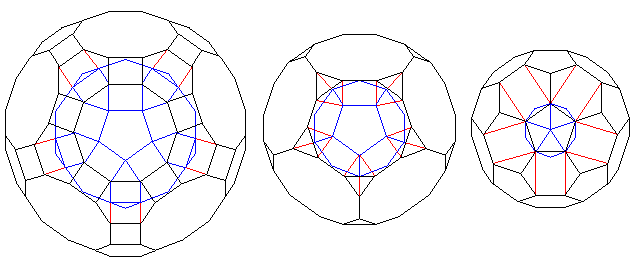Note how square prisms are formed in joining the small rhombicosidodecahedron and going to the great rhombicosidodecahedron, and that in the other cases, rectangles are formed joining corresponding edges of the solids involved.

We have seen above that the decagonal faces of the great rhombicosidodecahedron can be joined to the decagonal faces of the truncated dodecahedron by lines having the length of, and running in directions parallel to, the lines joining the vertices of the icosahedron to its center. This diagram: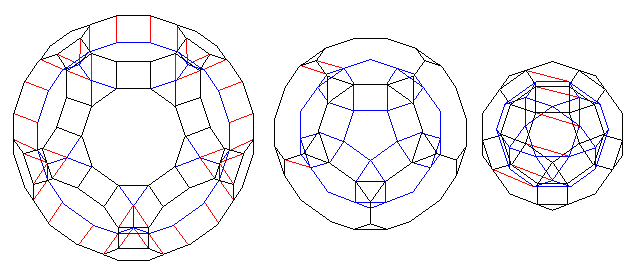shows that similar lines can join the decagonal faces of the truncated dodecahedron to internal decagons in the small rhombicosidodecahedron. And, in turn, those internal decagons can be joined to the decagons which divide an icosidodecahedron in half, but in this case, the lines are longer by a factor of phi.

Another symmetry results from the fact that it is possible to proceed from the small rhombicosidodecahedron to the dodecahedron using pentagonal prisms, as previously seen, and then, with a height multiplied by a factor of phi, from the pentagonal faces of the dodecahedron to the pentagons formed by five triangles of the icosahedron, and then, from these pentagons, using pentagonal prisms of the original height, to the pentagonal faces of the icosidodecahedron, and then, finally, again as previously seen, proceed from the pentagonal faces of the icosidodecahedron to the pentagonal faces of the truncated icosahedron with similar pentagonal prisms.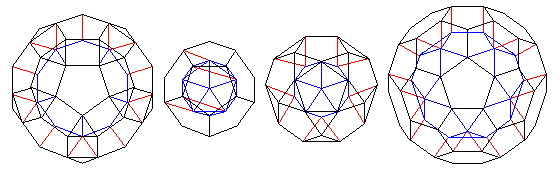[Next] [Up] [Previous]